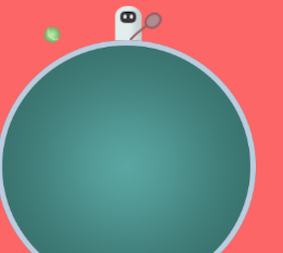# After colliding move the object back by the same circle trajectory

Hi, eguys. I am stuck with one feature and I have no idea how I can make it further. Would be much appreciated for the help or a hint.
So the point is, when the green ball(see attached screenshot) will collide with the racket, I want the ball to move backward(by the same trajectory). The ball and the racket have both defined Circle Colliders 2D.

Here is the code for my ball logic:

`````` public class BallMovement : MonoBehaviour {

private float RotateSpeed=1.3f;

private Vector2 _centre;
private float _angle;
private Vector2 offset;

void Start()
{
_centre = transform.position;
}

void Update()
{

_angle += Time.deltaTime * RotateSpeed;

offset = new Vector2 (-Mathf.Cos (_angle), Mathf.Sin (_angle)) * Radius;

transform.position = _centre + offset;

RotateSpeed+=acceleration;
if(RotateSpeed>maxSpeed){
RotateSpeed=maxSpeed;
}

void OnTriggerEnter2D(Collider2D other) {
if (other.gameObject.CompareTag("Racket")) {

// I thought I could make it in this way, but it is not working. And I think it is not the best approach at all
offset = new Vector2 (-Mathf.Cos (_angle), -Mathf.Sin (_angle)) * Radius;
}
}
}
``````

I attach here the screeshot for better visual understanding.Thanks.

You should remove - of your mathf.cos and sin.You should substract a value from your angle not make it negative.if you move with +1 angular speed in the first place and when you need to move reverse as you described you should move with -1 angular speed to change movement direction, so you should use this line of code `new Vector2(Mathf.Cos(_angle-YOURangularspeed,Mathf.Sin(_angle-YOURangularspeed)))`.